• 第16话：算法的空间复杂度
2021-02-04 08:32:18

类似于时间复杂度的讨论，一个算法的空间复杂度(Space Complexity)S(n)定义为该算法所耗费的存储空间，它也是问题规模n的函数。渐近空间复杂度也常常简称为空间复杂度。

空间复杂度(Space Complexity)是对一个算法在运行过程中临时占用存储空间大小的量度。一个算法在计算机存储器上所占用的存储空间，包括存储算法本身所占用的存储空间，算法的输入输出数据所占用的存储空间和算法在运行过程中临时占用的存储空间这三个方面。

我们在写代码时，完全可以用空间来换取时间，比如说，要判断某某年是不是闰年，你可能会花一点心思写了一个算法，而且由于是一个算法，也就意味着，每次给一个年份，都是要通过计算得到是否是闰年的结果。还有另一个办法就是，事先建立一个有2 050个元素的数组(年数略比现实多一点)，然后把所有的年份按下标的数字对应，如果是闰年，此数组项的值就是1，如果不是值为0。这样，所谓的判断某一年是否是闰年，就变成了查找这个数组的某一项的值是多少的问题。此时，我们的运算是最小化了，但是硬盘上或者内存中需要存储这2050个0和1。

算法的输入输出数据所占用的存储空间是由要解决的问题决定的，是通过参数表由调用函数传递而来的，它不随本算法的不同而改变。存储算法本身所占用的存储空间与算法书写的长短成正比，要压缩这方面的存储空间，就必须编写出较短的算法。算法在运行过程中临时占用的存储空间随算法的不同而异，有的算法只需要占用少量的临时工作单元，而且不随问题规模的大小而改变，我们称这种算法是“就地"进行的，是节省存储的算法，如这一节介绍过的几个算法都是如此；有的算法需要占用的临时工作单元数与解决问题的规模n有关，它随着n的增大而增大，当n较大时，将占用较多的存储单元，例如将快速排序和归并排序算法就属于这种情况。

通过一笔空间上的开销来换取计算时间的小技巧。到底哪一个好，其实要看你用在什么地方。

算法的空间复杂度通过计算算法所需的存储空间实现，算法空间复杂度的计算公式记作：S(n)= O(f(n))，其中，n为问题的规模，f(n)为语句关于n所占存储空间的函数。

一般情况下，一个程序在机器上执行时，除了需要存储程序本身的指令、常数、变量和输入数据外，还需要存储对数据操作的存储单元。若输入数据所占空间只取决于问题本身，和算法无关，这样只需要分析该算法在实现时所需的辅助单元即可。若算法执行时所需的辅助空间相对于输入数据量而言是个常数，则称此算法为原地工作，空间复杂度为O(1)。

关于O(1)的问题， O(1)是说数据规模和临时变量数目无关，并不是说仅仅定义一个临时变量。举例：无论数据规模多大，我都定义100个变量，这就叫做数据规模和临时变量数目无关。就是说空间复杂度是O(1)。

那就是指函数运行时处理数据的规模与空间和时间的一个变化时的比例关系，不是具体的数值。

当一个算法的空间复杂度为一个常量，即不随被处理数据量n的大小而改变时，可表示为O(1)；当一个算法的空间复杂度与以2为底的n的对数成正比时，可表示为0(10g2n)；当一个算法的空I司复杂度与n成线性比例关系时，可表示为0(n).若形参为数组，则只需要为它分配一个存储由实参传送来的一个地址指针的空间，即一个机器字长空间；若形参为引用方式，则也只需要为其分配存储一个地址的空间，用它来存储对应实参变量的地址，以便由系统自动引用实参变量。

通常，我们都使用“时间复杂度”来指运行时间的需求，使用“空间复杂度”指空间需求。当不用限定词地使用“复杂度”时，通常都是指时间复杂度。

对于一个算法，其时间复杂度和空间复杂度往往是相互影响的。当追求一个较好的时间复杂度时，可能会使空间复杂度的性能变差，即可能导致占用较多的存储空间；反之，求一个较好的空间复杂度时，可能会使时间复杂度的性能变差，即可能导致占用较长的运行时间。另外，算法的所有性能之间都存在着或多或少的相互影响。因此，当设计一个算法(特别是大型算法)时，要综合考虑算法的各项性能，算法的使用频率，算法处理的数据量的大小，算法描述语言的特性，算法运行的机器系统环境等各方面因素，才能够设计出比较好的算法。

延伸阅读

此文章所在专题列表如下：

更多相关内容
• 算法复杂度分为时间复杂度和空间复杂度。 其作用： 时间复杂度是指执行算法所需要的计算工作量； 而空间复杂度是指执行这个算法所需要的内存空间。 （算法的复杂性体现在运行该算法时的计算机所需资源的多少上，...
• 在编写Python中的自定义函数或算法时，减低时间复杂度和空间复杂度，会大大提升Python自定义函数或算法的整体性能。 时间复杂度 时间复杂度是用来估计自定义函数或算法运行时间的一个式子（单位）,时间复杂度常用“O...

# 简介

在编写Python中的自定义函数或算法时，减低时间复杂度空间复杂度，会大大提升Python自定义函数或算法的整体性能，提升性能的几个书写好习惯

• 尽量不要写循环，最好是一次就输出结果
• 如果写循环，尽量写一层循环就能有结果
• 避免嵌套

# 时间复杂度

时间复杂度是用来估计自定义函数或算法运行时间的一个式子（单位）,时间复杂度常用“O”表述，而且时间复杂度可被称为是渐近的，它考察当输入值大小趋近 ∞ \infin 时的情况

时间复杂度为 O(1)的例子

print('Hello!')
print(1)


时间复杂度为 O(n)的例子

# 其中range(n) 换成range(length(n))或者直接换成n，其时间复杂度都为 O(n)
for i in range(n):
print('Hello!')


时间复杂度为O(n^2)的例子

# 其中range(n) 换成range(length(n))或者直接换成n，其时间复杂度都为 O(n^2)
for i in range(n):
for j in range(n):
print('Hello!')

for i in range(n):
print('Hello!')
for j in range(n):
print('Hello!')

for i in range(n):
for j in range(i):
print('Hello!')


时间复杂度为O(n^3)的例子

# 其中range(n) 换成range(length(n))或者直接换成n，其时间复杂度都为 O(n^3)
for i in range(n):
for j in range(n):
for k in range(n):
print('Hello!')


由上述时间复杂度O(n)的变化可以得出这样的结论：O(n)中n的几次方取决于自定义函数或算法中存在几个循环

时间复杂度为O(n^n)的例子

# 其中range(n) 换成range(length(n))或者直接换成n，其时间复杂度都为 O(n^n)
for i in range(n):
for j in range(n):
for k in range(n):
for l in range(n):
.
.
.
for o in range(n):
print('Hello!')


时间复杂度为O( l o g 2 n log_2n )的例子

n = 128
while n > 1:
print(n)
n = n // 2 # //表示整除法


计算上述问题的时间复杂度步骤：

1. 2 7 = 128 2^7 = 128
2. l o g 2 128 = 7 log_2128 = 7
3. 循环减半的时间复杂度为O( l o g 2 128 log_2128 )，即O( l o g n 128 log_n128 ) = O(7)

复杂度为O( l o g 2 n log_2n )

n = n
while n > 1:
print(n)
n = n // 2 # //表示整除法


常见的时间复杂度高低排序：O(1)<O( l o g n log_n )<O(n)<O( n l o g n nlog_n )<O( n 2 n^2 )<O( n 2 l o g n n^2log_n )<O( n 3 n^3 )

# 空间复杂度

空间复杂度：用来评估自定义函数或算法内存占用大小

空间复杂度为 1的例子

p = 'Python'
l = 'like'
m = 'me'


空间复杂度为 n

p = [1,2,3,....,n]


空间复杂度为 n*n

p_n = [[1,2,3,....,n],[1,2,3,....,n],.....,[1,2,3,....,n]]


空间复杂度为 n * n * n

p_n = [[[1,2,3,....,n],[1,2,3,....,n],.....,[1,2,3,....,n]],
[[1,2,3,....,n],[1,2,3,....,n],.....,[1,2,3,....,n]],
....,
[[1,2,3,....,n],[1,2,3,....,n],.....,[1,2,3,....,n]]


空间复杂度却决于：

• 变量的长度
• 变量的个数
• 变量的复杂程度

# 示例展示

自定义方差（variance）函数简化，以及计算其对应的时间复杂度和空间复杂度

def variance (*args): # *args不限定输入的可变量，这里输入的数量为n
total = 0 #时间复杂度为 1；空间复杂度为 1
average = float(sum(args))/len(args)
# 时间复杂度为 n+1 其中sum（args）为n，len(args)为 1
# 空间复杂度为 1
for val in args:
total +=(val-average)**2
# 时间复杂度为 n，即进行了n次循环
# 空间复杂度为 n，即存储了n次total


时间复杂度：1+n+1+n+1 = 2n+1
空间复杂度：1+1+n+1 = n+3

展开全文• 算法空间复杂度详细介绍

上一篇博客中我们学习了时间复杂度：需要的小伙伴请点击：

https://blog.csdn.net/qq_43355165/article/details/122644530

# 概念定义

空间复杂度涉及的空间类型有：

• 输入空间：存储输入数据所需的空间大小；
• 暂存空间： 算法运行过程中，存储所有中间变量和对象等数据所需的空间大小；
• 输出空间： 算法运行返回时，存储输出数据所需的空间大小；
通常情况下，空间复杂度指在输入数据大小为N时，算法运行所使用的暂存空间 + 输出空间的总体大小。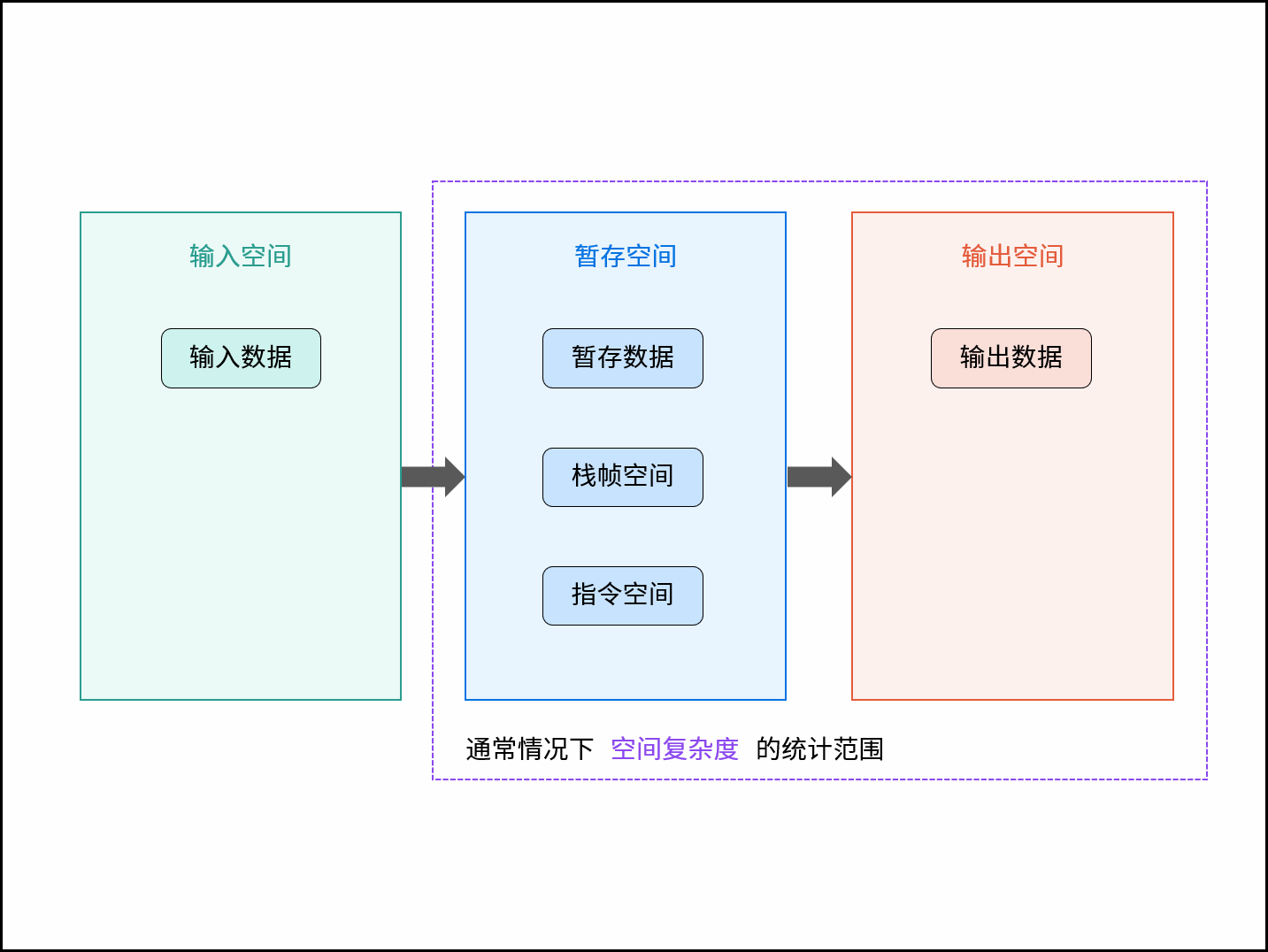而根据不同来源，算法使用的内存空间分为三类：

## 指令空间：

编译后，程序指令所使用的内存空间。

## 数据空间：

算法中的各项变量使用，包括：声明的变量、变量、动态数组、动态对象等使用的内存空间。

class Node:
def __init__(self, val):
self.val = val
self.next = None
def algorithm(N):
num = N        # 数组
nums =  * N # 动态数组
node = Node(N) # 动态对象


## 栈帧空间：

程序调用函数是基于栈实现的，函数在调用期间，占用常量大小的栈帧空间，直至返回后释放。如以下代码所示，在循环中调用函数，每轮调用test()返回后，栈帧空间已被释放，因此空间复杂度仍为O(1)。

def test():
return 0
def algorithm(N):
for _ in range(N):
test()


算法中，栈帧空间的累计常出现于递归调用。如以下代码所示，通过递归调用，会同时存在N个未返回的函数 algorithm()，此时累计使用O(N)大小的栈帧空间。

def algorithm(N):
if N <= 1: return 1
return algorithm(N - 1) + 1


# 符号表示

通常情况下，空间复杂度统计算法在“最差情况”下使用的空间大小，以体现算法运行所需预留的空间量，使用符号O表示。
最差情况有两层含义，分别为最差输入数据、算法运行中的最差运行点。例如以下代码：

• 最差输入数据：当N<=10时，数组nums的长度恒定为10，空间复杂度为O(10) = O(1)；当N > 10时，数组nums长度为N，空间复杂度为O(N)；因此，空间复杂度应为最差输入数据情况下的O(N)。
• 最差运行点：在执行nums =  * 10时，算法仅使用O(1)大小的空间；而当执行nums =  * N时，算法使用O (N)的空间；因此，空间复杂度应为最差运行点的O(N)。
def algorithm(N):
num = 5			   # O(1)
nums =  * 10    # O(1)
if N > 10:
nums =  * N # O(N)


# 常见种类

根据从小到大排列，常见的算法空间复杂度有：
O(1) < O(logN) < O(N) < O(N 2) < O(2N)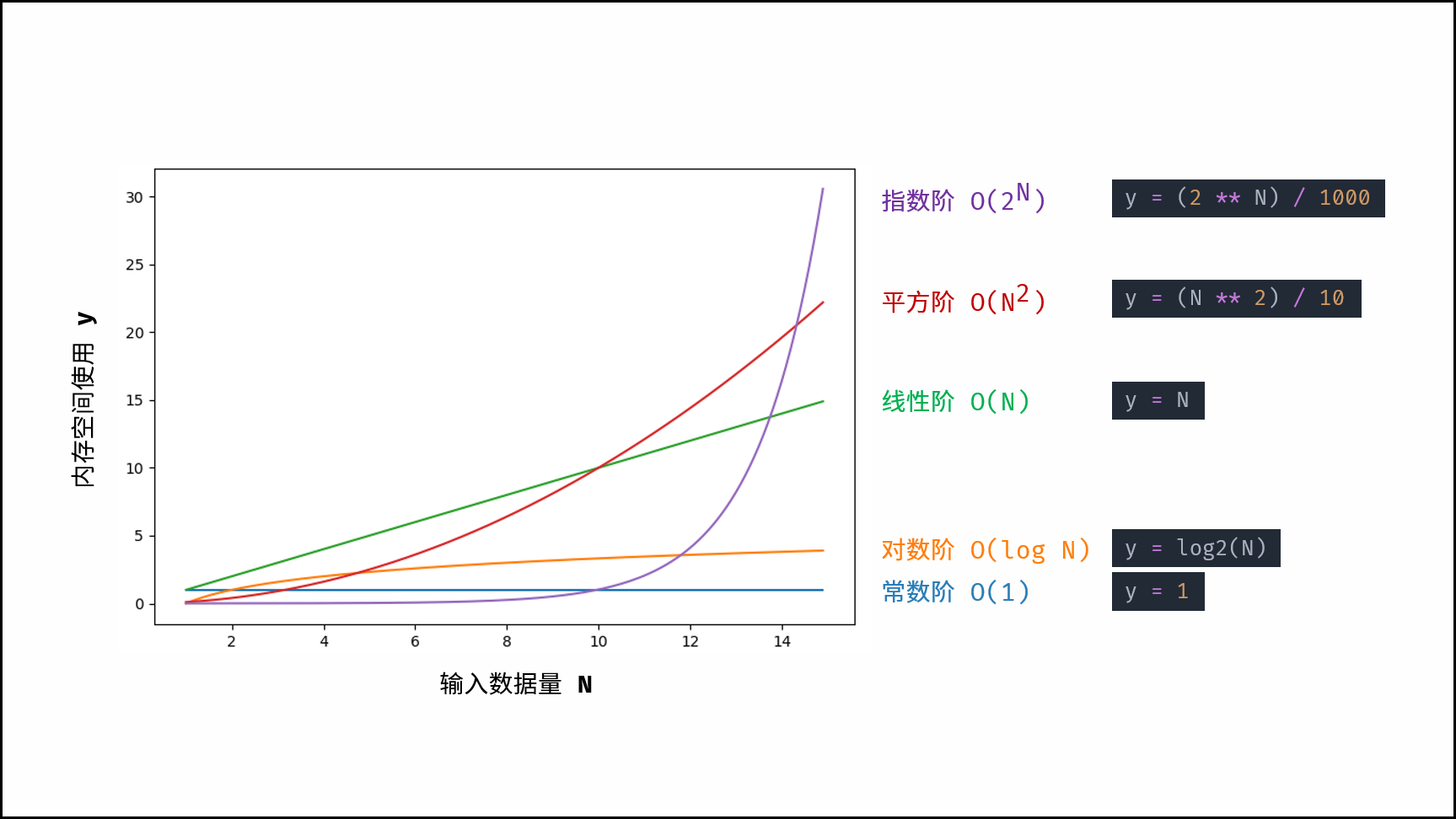# 示例解析

对于以下所有示例，设输入数据大小为正整数N，节点类Node、函数test()如以下代码所示。

class Node:
def __init__(self, val):
self.val = val
self.next = Node
# 函数 test()
def test():
return 0


## 常数O(1):

普通常量、变量、对象、元素数量与输入数据大小N无关的集合，皆使用常数大小的空间。

def algorithm(N):
num = 0
nums =  * 10000
node = Node(0)
dic = {0: '0'}


如以下代码所示，虽然函数test()调用了N次，但每轮调用后test()已返回，无累计栈帧空间使用，因此空间复杂度仍为O(1)。

def algorithm(N)
for _ in range(N):
test()


## 线性O(N)

元素数量与N呈线性关系的任意类型集合（常见于一维数组、链表、哈希表等），皆使用线性大小的空间。

def algorithm(N):
nums_1 =  * N
nums_2 =  * (N // 2)

nodes = [Node(i) for i in range(N)]

dic = {}
for i in range(N):
dic[i] = str(i)


如下图与代码所示，此递归调用期间，会同时存在N个未返回的algorithm()函数，因此使用O(N)大小的栈帧空间。

def algorithm(N):
if N <= 1: return 1
return algorithm(N - 1) + 1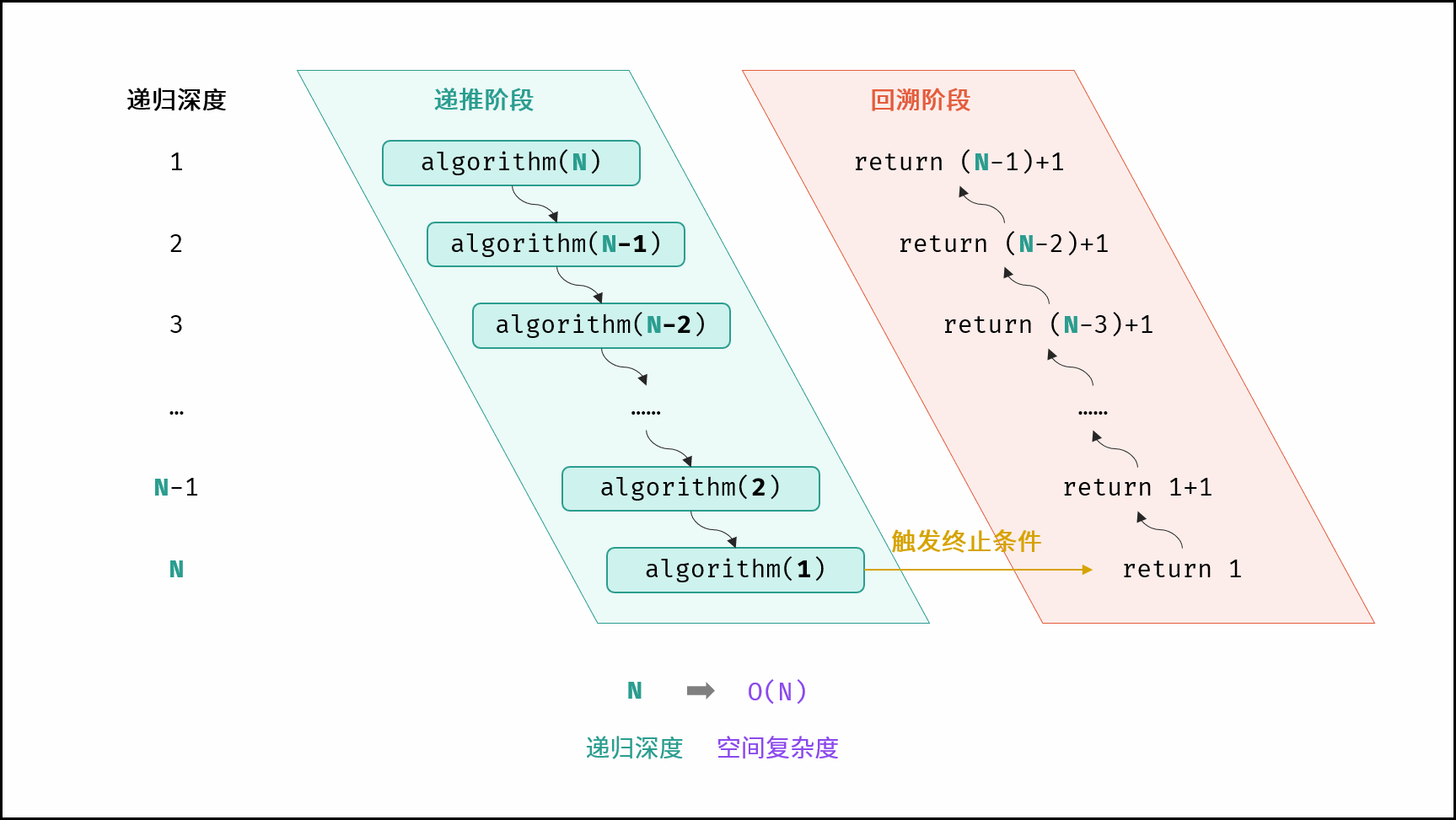## 平方O(N2)：

元素数量与N呈平方关系的任意类型集合（常见于矩阵），皆使用平方大小的空间。

def algorithm(N):
num_matrix = [[0 for j in range(N)] for i in range(i)]
node_matrix = [[Node(j) for j in range(N)] for i in range(N)]


如下图与代码所示，递归调用时同时存在 N 个未返回的algorithm()函数，使用 O(N) 栈帧空间；每层递归函数中声明了数组，平均长度为N/2 ，使用 O(N)空间；因此总体空间复杂度为 O(N2)。

def algorithm(N):
if N <= 0: return 0
nums =  * N      # O(N)
return algorithm(N - 1)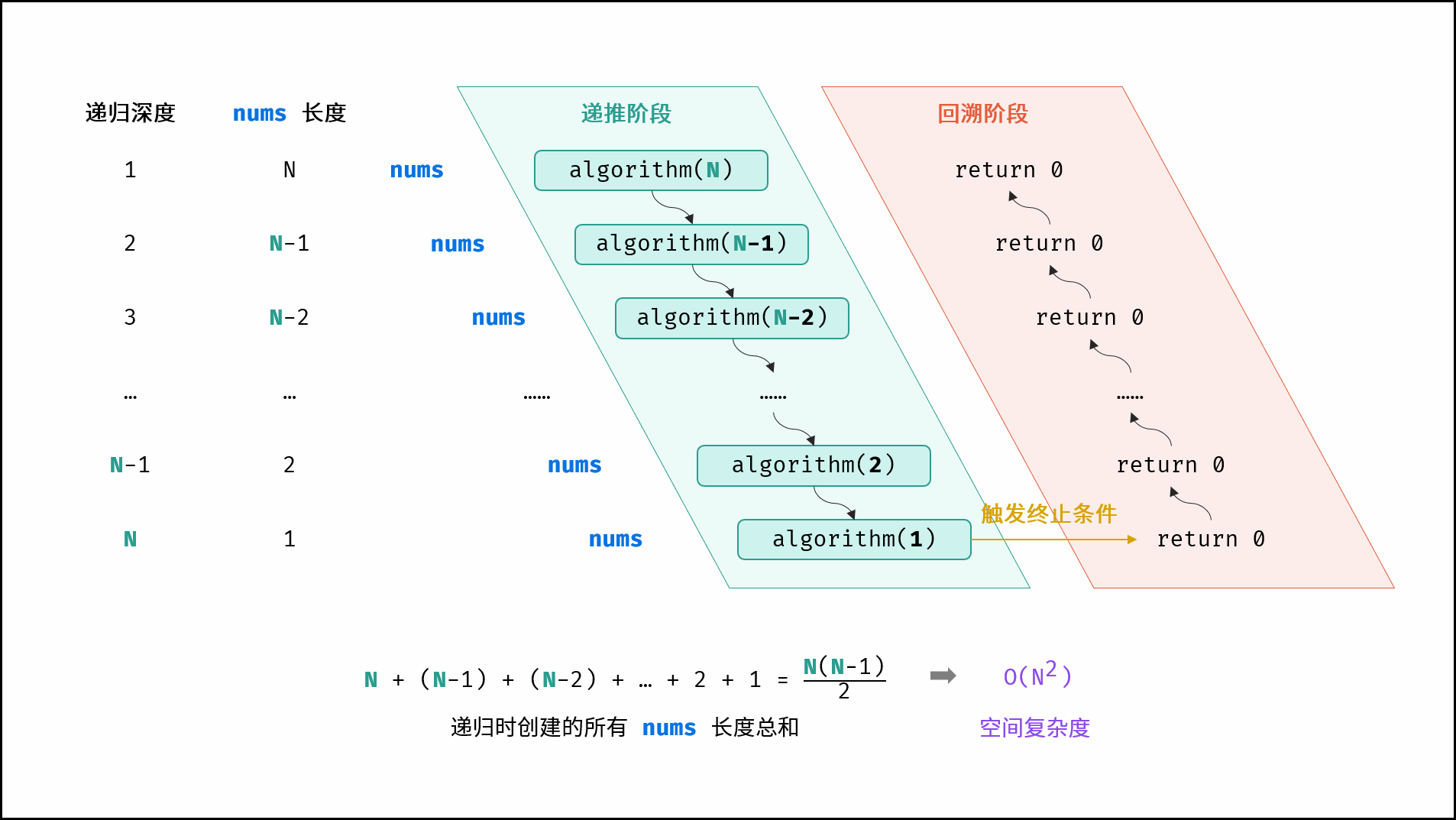## 指数O(2N) ：

指数阶常见于二叉树、多叉树。例如，高度为N满二叉树的节点数量为2N，占用O(2N)大小的空间；同理，高度为N满m叉树的节点数量为mN，占用O(mN) = O(2N)大小的空间。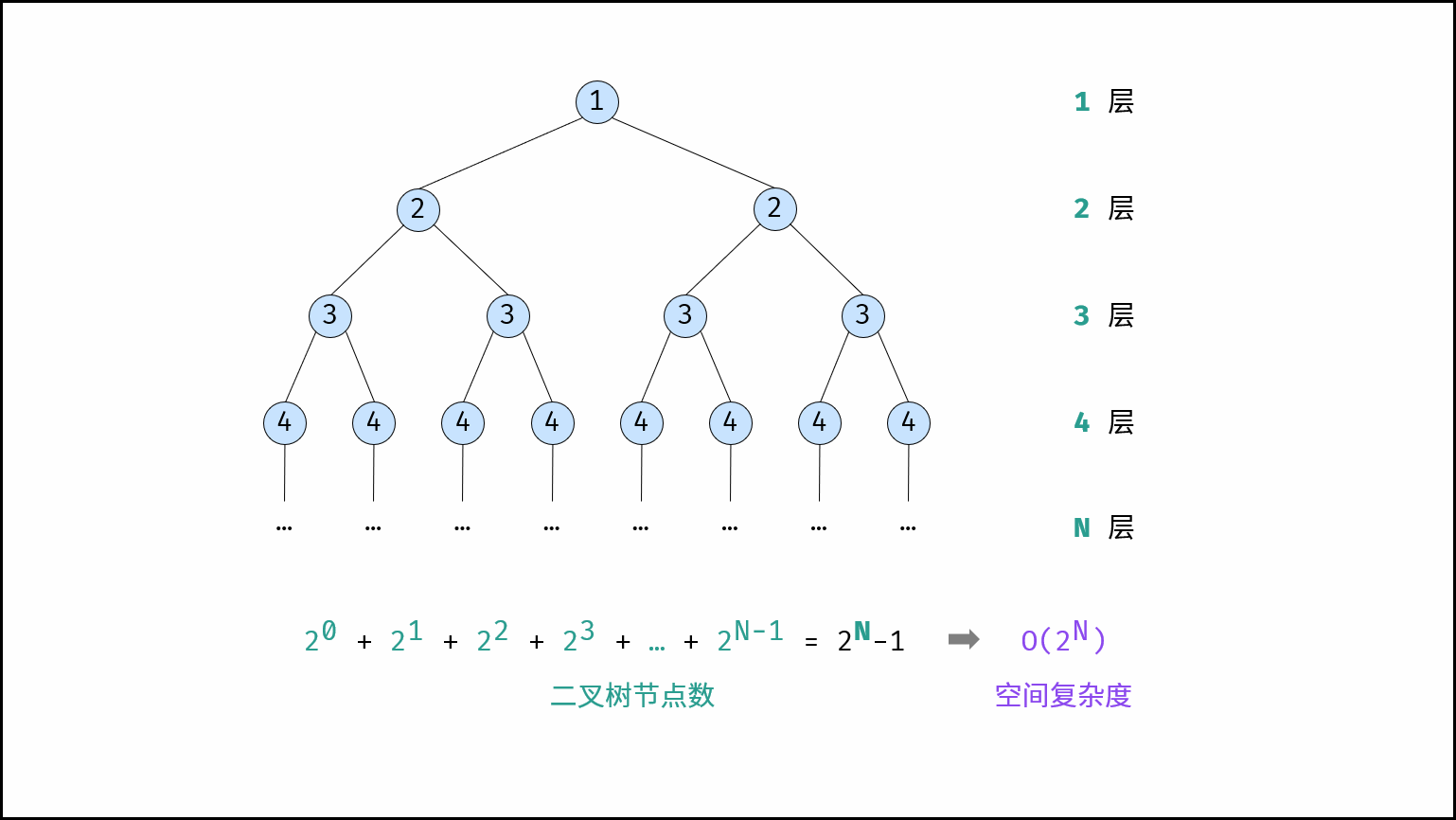## 对数O(logN) ：

对数阶常出现于分治算法的栈帧空间累计、数据类型转换等，例如：

• 快速排序 ，平均空间复杂度为 Θ(logN) ，最差空间复杂度为 O(N) 。拓展知识：通过应用 Tail Call Optimization ，可以将快速排序的最差空间复杂度限定至O(N)
• 数字转化为字符串 ，设某正整数为 N ，则字符串的空间复杂度为 O(logN) 。推导如下：正整数 N 的位数为 log10N ，即转化的字符串长度为log10N，因此空间复杂度为 O(logN)

## 时空权衡

对于算法的性能，需要从时间和空间的使用情况来综合评价。优良的算法应具备两个特性，即时间和空间复杂度皆较低。而实际上，对于某个算法问题，同时优化时间复杂度和空间复杂度是非常困难的。降低时间复杂度，往往是以提升空间复杂度为代价的，反之亦然。

由于当代计算机的内存充足，通常情况下，算法设计中一般会采取「空间换时间」的做法，即牺牲部分计算机存储空间，来提升算法的运行速度。

以 LeetCode 全站第一题 两数之和 为例，「暴力枚举」和「辅助哈希表」分别为「空间最优」和「时间最优」的两种算法。

### 方法一：暴力枚举

时间复杂度 O(N2) ，空间复杂度O(1) ；属于「时间换空间」，虽然仅使用常数大小的额外空间，但运行速度过慢。

class Solution:
def twoSum(self, nums: List[int], target: int) -> List[int]:
for i in range(len(nums) - 1):
for j in range(i + 1, len(nums)):
if nums[i] + nums[j] == target:
return i, j
return


### 方法二：辅助哈希表

时间复杂度 O(N)，空间复杂度 O(N)；属于「空间换时间」，借助辅助哈希表 dic ，通过保存数组元素值与索引的映射来提升算法运行效率，是本题的最佳解法。

class Solution:
def twoSum(self, nums: List[int], target: int) -> List[int]:
dic = {}
for i in range(len(nums)):
if target - nums[i] in dic:
return dic[target - nums[i]], i
dic[nums[i]] = i
return []


在 LeetCode 题目中，「输入空间」和「输出空间」往往是固定的，是必须使用的内存空间。因希望专注于算法性能对比，本 LeetBook 的题目解析的空间复杂度仅统计「暂存空间」大小。

展开全文算法 链表 数据结构 leetcode
• 空间复杂度的分析只要计算出是否持续的申请内存就好，常见的空间复杂度是O（1）、O（n）、O（n^2）。越高阶复杂度的算法，执行效率越低，从小到大依次是：O（1）、O（logn）、O（n）、o（nlogn）、o（n^2) ,对应图像...

B 先引入一段代码：

1 int cal(intn) {2 int ret = 0;3 int i = 1;4 for (; i < n; ++i) {5 ret = ret +f(i);6 }7 }8

9 int f(intn) {10 int sum = 0;11 int i = 1;12 for (; i < n; ++i) {13 sum = sum +i;14 }15 returnsum;16 }

对于cal函数，只看执行次数最多的4～6行代码，负责一共执行了2n次，可对于f函数内部也执行了2n次，那么总的时间复杂度就是：T(n)= O(cal(n)* f (n)= O(4n^2)= O(n^2)。

时间和空间复杂度用来度量程序的运行时间效率和占用空间大小，即大O表示法：T(n) = O(f(n))，最终求得的时间复杂度，需要省略掉表达式中的系数、低阶、常量，因为我们只关心占用时间和空间最大的那一部分代码。

几种常见的时间复杂度量级：这里主要分析一下对数阶复杂度，这样一段代码：

1 i=1;2 while (i <=n) {3 i = i * 2;4 }

变量i从1开始取值，每次循环乘以2，得出2^x=n，根据对数公式，x = log2^n，忽略掉对数底，则时间复杂度为O(logn)。

时间复杂度的全称是渐进时间复杂度，表示算法的执行时间与数据规模之间的增长关系，那么空间复杂度就是表示算法的存储空间和数据规模之间的存储关系。空间复杂度的分析只要计算出是否持续的申请内存就好，常见的空间复杂度是O(1)、O(n)、O(n^2)。越高阶复杂度的算法，执行效率越低，从小到大依次是：O(1)、O(logn)、O(n)、o(nlogn)、o(n^2) ,对应图像：展开全文• 1.3 空间复杂度 1.4 递归 1.5 汉诺塔问题 数据结构与算法(Python版) # 1. 入门 1.1 算法概念 概念： 算法就是一个计算过程，解决问题的方法 程序 = 数据结构 + 算法 1.2 时间复杂度 时间复杂度是用来...数据结构 算法
• 时间复杂度用于度量算法的计算工作量，空间复杂度用于度量算法占用的内存空间。这篇文章主要介绍了Python算法中的时间复杂度,需要的朋友可以参考下
• Python与时间复杂度Python内置性能分析timeit模块list内置操作的时间复杂度dict内置函数复杂度 Python内置性能分析 函数是对基本操作的封装，不能直接将函数看为一个基本操作！ timeit模块 from timeit import ...算法
• leetcode怎么计算空间复杂度是指 面试题记录 [toc] 目录 - - - - - - - - - - - - - python 基础 装饰器（什么是AOP/面向切面编程）, python代码执行原理， pthon的int怎么实现的 ，is和==的区别，深拷贝-浅拷贝，...
• 我之前写了两篇关于时间复杂度的文章，分别是究竟什么...空间复杂度是对一个算法在运行过程中占用内存空间大小的量度，记做S(n)=O(f(n))。利用程序的空间复杂度，可以对程序运行时所需要多少内存有个预先估计。我这...
• 这是一个系列篇，主要是用python刷题，适用于喜欢用python刷题的小伙伴一起交流和讨论，之前作者基于java、c\c++都刷过，这次使用python更加系统的根据校招高频题进行分析。 首先分析一下做题需要注意的重点内容： ...leetcode刷题 算法
• 算法(algorithm)本质上是一连串的计算。同一个问题可以使用不同算法解决，但计算过程中消耗的时间和资源可能...空间维度就是算法需要占用的内存空间，空间复杂度(space complexity)是常用分析单位。因此，分析算法...
• 1、啥是算法：算法就是一个计算过程，解决问题的方法 2、算法的特征： 有穷性：算法必须在有限步骤内终止 确切性：算法的每一步需要有确切的步骤 输入项：一个算法有0个或多个输入，以刻画运算对象的初始情况，...
• 算法的时间复杂度是一个函数，它定量描述了该算法的运行时间，时间复杂度常用“O”表述，使用这种方式时，时间复杂度可被称为是渐近的，它考察当输入值大小趋近无穷时的情况时间复杂度是用来估计算法运行时间的一个...
• 时间复杂度执行算法所需的计算工作量。一般来说，计算机算法是问题规模n的函数f(n),算法的时间复杂度也因此记做T(n)=O(f(n));常见时间复杂度有:常数阶、线性阶、平方阶、立方阶、对数阶、nlog2n阶、指数阶效率：O(1)...
• 2. 算法复杂度包括： 时间复杂度、空间复杂度 3. 时间复杂度：即算法的执行效率，是指算法的执行时间与算法的输入值之间的关系。一般用大O表示。主要关注for\while循环。写代码要考虑如何减少循环 def test1(n): ...
• leetcode怎么计算空间复杂度是指 LeetCode 题解 Python3 # Title Solution Times 1438 3 1143 2 1047 3 1046 1 1011 1 1006 1 1004 3 995 3 830 2 765 2 706 1 705 1 697 1 684 2 605 3 566 1 561 1 547 2 509 1 503...
• python学习：算法和时间复杂度算法什么是算法？算法(Algorithm)是指解题方案的准确而完整的描述，是一系列解决问题的清晰指令，算法代表着用系统的方法描述解决问题的策略机制。算法也可以说是我们为了解决问题而...
• 常见的时间复杂度高低排序： O(1)<O(logn)<O(n)<O(nlogn)<O(n²)<O(n²logn)<O(n³)<O(2ⁿ)<O(n!)<O(nⁿ) 列表（List）： python的列表内部实现是数组（具体实现要看解析器），因此就...时间
•   我们前面讲过list、deque、堆、字典树等高性能计算的技巧，这一节我们来整理一下Python中常用操作的时间复杂度。本文中的N表示容器的元素数量，K表示参数中元素的数量或参数的值。 list lst = list(range(10,...
• 该时间复杂度计算基于当前(译注：至少是2011年之前)的CPython实现。其他Python的实现(包括老版本或者尚在开发的CPython实现)可能会在性能表现上有些许小小的差异，但一般不超过一个O(log n)...
• 内排序有可以分为以下几类：（1）插入排序：直接插入排序、二分法插入排序、希尔排序（2）选择排序：直接选择排序、堆排序（3）交换排序：冒泡排序、快速排序（4）归并排序（5）基数排序排序方法时间复杂度(平均)...
• 文章目录最坏时间复杂度时间复杂度的几条基本计算规则常见时间复杂度的关系Python内置类型性能分析timeit模块list 内置操作的时间复杂度dict内置操作的实践复杂度 最坏时间复杂度 分析算法时，存在几种可能的考虑: ...数据结构 算法
• 时间复杂度是同一问题可用不同算法解决，而一个算法的质量优劣将影响到算法乃至程序的效率。算法分析的目的在于选择合适算法和改进算法。计算机科学中，算法的时间复杂度是一个函数，它定性描述了该...定义在计算...
• print(time()-t) 2.76320481300354 >>> 那么我们要用什么方式去衡量一个算法的好坏，所以我们引进了时间复杂度和空间复杂度。 时间复杂度主要衡量一个算法的运行快慢，而空间复杂度主要衡量一个算法运行所占用空间。...算法 c++ 斐波那契数列...